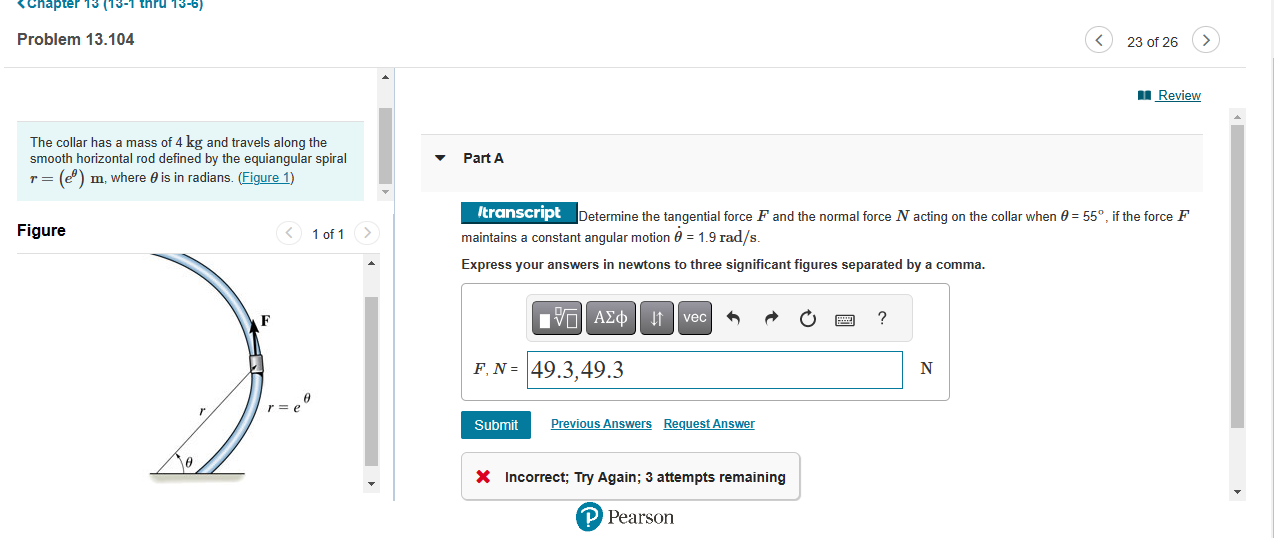Home / Expert Answers / Mechanical Engineering / the-collar-has-a-mass-of-4kg-and-travels-along-the-smooth-horizontal-rod-defined-by-the-equiangu-pa787

# (Solved): The collar has a mass of 4kg and travels along the smooth horizontal rod defined by the equiangu ...The collar has a mass of and travels along the smooth horizontal rod defined by the equiangular spiral , where is in radians. Determine the tangential force and the normal force acting on the collar when , if the force maintains a constant angular motion . Express your answers in newtons to three significant figures separated by a comma. Incorrect; Try Again; 3 attempts remaining

We have an Answer from Expert